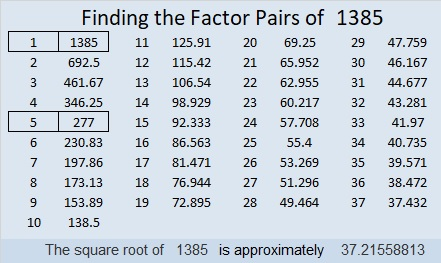# 1385 Mystery Level

You can suspect that the common factor of 9 and 6 is either 1 or 3, but don’t jump to conclusions about which one will satisfy this mystery! There’s important evidence elsewhere in the puzzle that you should consider first.Print the puzzles or type the solution in this excel file: 10 Factors 1373-1388

Now I’ll share some facts about the puzzle number, 1385:

• 1385 is a composite number.
• Prime factorization: 1385 = 5 × 277
• 1385 has no exponents greater than 1 in its prime factorization, so √1385 cannot be simplified.
• The exponents in the prime factorization are 1, and 1. Adding one to each exponent and multiplying we get (1 + 1)(1 + 1) = 2 × 2 = 4. Therefore 1385 has exactly 4 factors.
• The factors of 1385 are outlined with their factor pair partners in the graphic below.1385 is the sum of two squares in two different ways:
32² + 19² = 1385
37² + 4² = 1385

1385 is the hypotenuse of a Pythagorean triple:
296-1353-1385 calculated from 2(37)(4), 37² – 4², 37² + 4²
575-1260-1385 which is 5 times (115-252-277)
663-1216-1385 calculated from 32² – 19², 2(32)(19), 32² + 19²
831-1108-1385 which is (3-4-5) times 277

This site uses Akismet to reduce spam. Learn how your comment data is processed.